# Productive Rage

Dan's techie ramblings

## How are barcodes read?? (Library-less image processing in C#)

I've been using MyFitnessPal and it has the facility to load nutrition information by scanning the barcode on the product. I can guess how the retrieval works once the barcode number is obtained (a big database somewhere) but it struck me that I had no idea how the reading of the barcode itself worked and.. well, I'm curious and enjoy the opportunity to learn something new to me by writing the code to do it. I do enjoy being able to look up (almost) anything on the internet to find out how it works!

(For anyone who wants to either play along but not copy-paste the code themselves or for anyone who wants to jump to the end result, I've put the code - along with the example image I've used in this post - up on a GitHub repo)

### The plan of attack

There are two steps required here:

1. Read image and try to identify areas that look like barcodes
2. Try to extract numbers from the looks-like-a-barcode regions

As with anything, these steps may be broken down into smaller tasks. The first step can be done like this:

1. Barcodes are black and white regions that have content that has steep "gradients" in image intensity horizontally (where there is a change from a black bar to a white space) and little change in intensity vertically (as each bar is a vertical line), so first we greyscale the image and then generate horizontal and vertical intensity gradients values for each point in the image and combine the values by subtracting vertical gradient from horizontal gradient
2. These values are normalised so that they are all on the scale zero to one - this data could be portrayed as another greyscale image where the brightest parts are most likely to be within barcodes
3. These values are then "spread out" or "blurred" and then a threshold value is applied where every value about it is changed into a 1 and every value below it a 0
4. This "mask" (where every value is a 0 or 1) should have identified many of the pixels within the barcodes and we want to group these pixels into distinct objects
5. There is a chance, though, that there could be gaps between bars that mean that a single barcode is spread across multiple masked-out objects and we need to try to piece them back together into one area (since the bars are tall and narrow, this may be done by considering a square area over every object and then combining objects whose squared areas overlap into one)
6. This process will result in a list of areas that may be barcodes - any that are taller than they are wide are ignored (because barcode regions are always wider than they are tall)

The second step can be broken down into:

1. Take the maybe-barcode region of the image, greyscale it and then turn into a mask by setting any pixel with an intensity less than a particular threshold to zero and otherwise to one
2. Take a horizontal slice across the image region - all of the pixels on the first row of the image - and change the zero-or-one raw data into a list of line lengths where a new line starts at any transition from zero-to-one or one-to-zero (so "01001000110" becomes "1,1,2,1,3,2,1" because there is 1x zero and then 1x one and then 2x zero and then 1x one, etc..)
3. These line lengths should correspond to bar sizes (and space-between-bar sizes) if we've found a barcode - so run the values through the magic barcode bar-size-reading algorithm (see section 2.1 in https://www.ncbi.nlm.nih.gov/pmc/articles/PMC2859730/) and if we get a number (and the checksum is correct) then we're done, hurrah!
4. If we couldn't get a number from this horizontal slice then move one pixel down and go back around
5. If it was not possible to extract a number from any of the slices through the image region then it's either not a barcode or it's somehow so distorted in the image that we can't read it

This approach is fairly resilient to changes in lighting and orientation because the barcode regions are still likely to have the highest horizontal intensity gradient whether the image is dark or light (and even if part of the image is light and part of it is dark) and the barcode-reading algorithm works on ratios of bar/space-between-bar widths and these remain constant if the image is rotated.

(Some of the techniques are similar to things that I did in my Face or no face (finding faces in photos using C# and Accord.NET) and so I'll be using some of the same code shortly that I described then)

### Identifying maybe-barcode images

Let's this image as an example to work with (peanut butter.. I do love peanut butter) -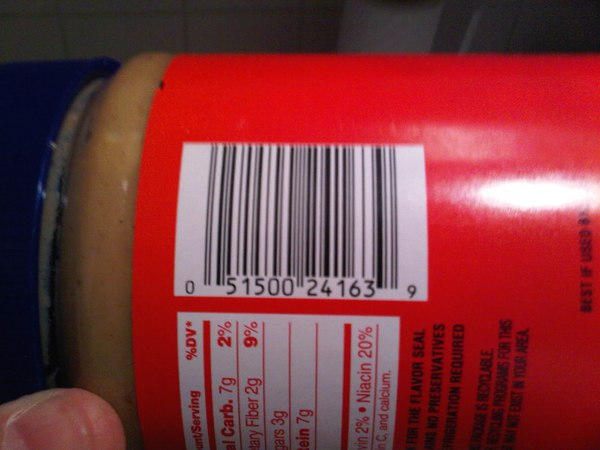Before looking at any code, let's visualise the process.

We're going to consider horizontal and vertical gradient intensity maps - at every point in the image we either look to the pixels to the left and to the right (for the horizontal gradient) or we look at the pixels above and below (for the vertical gradient) and the larger the change, the brighter the pixel in the gradient intensity map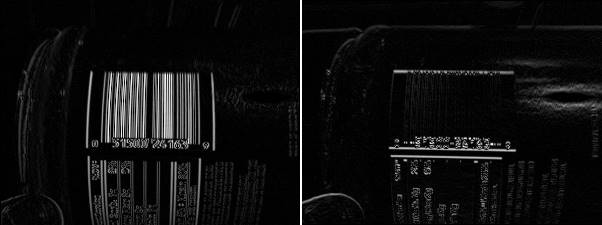And when they're combined by subtracting the vertical gradient at each point from the horizontal gradient, it looks lke this: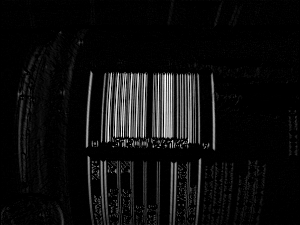If this image is blurred then we get this: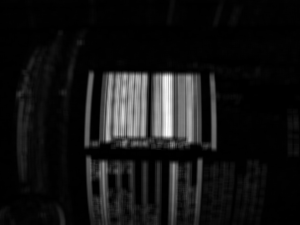.. and if we create a binary mask by saying "normalise the intensity values so that their range goes from zero (for the darkest pixel) to one (for the brightest) and then set any pixels that are in the bottom third in terms of intensity to 0 and set the rest to 1" then we get this: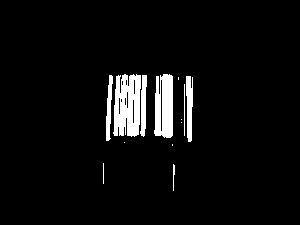If each distinct area (where an "area" means "a group of pixels that are connected") is identified and squares overlaid and centered around the areas then we see this: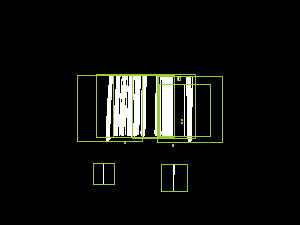.. and if the areas whose bounding squares overlap are combined and then cropped around the white pixels then we end up with this: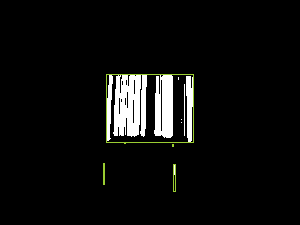This has identified the area around the barcode and also two tiny other areas - when we come to trying to read barcode numbers out of these, the tiny regions will result in no value while the area around the genuine barcode content should result in a number successfully being read. But I'm getting ahead of myself.. let's look at the code required to perform the above transformations.

``````public static class DataRectangle
{
public static DataRectangle<T> For<T>(T[,] values) => new DataRectangle<T>(values);
}

public sealed class DataRectangle<T>
{
public DataRectangle(T[,] values) : this(values, isolationCopyMayBeBypassed: false) { }
private DataRectangle(T[,] values, bool isolationCopyMayBeBypassed)
{
if ((values.GetLowerBound(0) != 0) || (values.GetLowerBound(1) != 0))
throw new ArgumentException("Both dimensions must have lower bound zero");
var arrayWidth = values.GetUpperBound(0) + 1;
var arrayHeight = values.GetUpperBound(1) + 1;
if ((arrayWidth == 0) || (arrayHeight == 0))
throw new ArgumentException("zero element arrays are not supported");

Width = arrayWidth;
Height = arrayHeight;

if (isolationCopyMayBeBypassed)
_protectedValues = values;
else
{
_protectedValues = new T[Width, Height];
Array.Copy(values, _protectedValues, Width * Height);
}
}

/// <summary>
/// This will always be greater than zero
/// </summary>
public int Width { get; }

/// <summary>
/// This will always be greater than zero
/// </summary>
public int Height { get; }

public T this[int x, int y]
{
get
{
if ((x < 0) || (x >= Width))
throw new ArgumentOutOfRangeException(nameof(x));
if ((y < 0) || (y >= Height))
throw new ArgumentOutOfRangeException(nameof(y));
return _protectedValues[x, y];
}
}

public IEnumerable<Tuple<Point, T>> Enumerate(Func<Point, T, bool>? optionalFilter = null)
{
for (var x = 0; x < Width; x++)
{
for (var y = 0; y < Height; y++)
{
var value = _protectedValues[x, y];
var point = new Point(x, y);
if (optionalFilter?.Invoke(point, value) ?? true)
yield return Tuple.Create(point, value);
}
}
}

public DataRectangle<TResult> Transform<TResult>(Func<T, TResult> transformer)
{
return Transform((value, coordinates) => transformer(value));
}

public DataRectangle<TResult> Transform<TResult>(Func<T, Point, TResult> transformer)
{
var transformed = new TResult[Width, Height];
for (var x = 0; x < Width; x++)
{
for (var y = 0; y < Height; y++)
transformed[x, y] = transformer(_protectedValues[x, y], new Point(x, y));
}
return new DataRectangle<TResult>(transformed, isolationCopyMayBeBypassed: true);
}
}
``````

And then I'm going to add a way to load image data into this structure -

``````public static class BitmapExtensions
{
/// <summary>
/// This will return values in the range 0-255 (inclusive)
/// </summary>
// Based on http://stackoverflow.com/a/4748383/3813189
public static DataRectangle<double> GetGreyscale(this Bitmap image)
{
var values = new double[image.Width, image.Height];
var data = image.LockBits(
new Rectangle(0, 0, image.Width, image.Height),
PixelFormat.Format24bppRgb
);
try
{
var pixelData = new Byte[data.Stride];
for (var lineIndex = 0; lineIndex < data.Height; lineIndex++)
{
Marshal.Copy(
source: data.Scan0 + (lineIndex * data.Stride),
destination: pixelData,
startIndex: 0,
length: data.Stride
);
for (var pixelOffset = 0; pixelOffset < data.Width; pixelOffset++)
{
// Note: PixelFormat.Format24bppRgb means the data is stored in memory as BGR
const int PixelWidth = 3;
var r = pixelData[pixelOffset * PixelWidth + 2];
var g = pixelData[pixelOffset * PixelWidth + 1];
var b = pixelData[pixelOffset * PixelWidth];
values[pixelOffset, lineIndex] = (0.2989 * r) + (0.5870 * g) + (0.1140 * b);
}
}
}
finally
{
image.UnlockBits(data);
}
return DataRectangle.For(values);
}
}
``````

``````private static IEnumerable<Rectangle> GetPossibleBarcodeAreasForBitmap(Bitmap image)
{
var greyScaleImageData = image.GetGreyscale();
var combinedGradients = greyScaleImageData.Transform((intensity, pos) =>
{
// Consider gradients to be zero at the edges of the image because there aren't pixels
// both left/right or above/below and so it's not possible to calculate a real value
var horizontalChange = (pos.X == 0) || (pos.X == greyScaleImageData.Width - 1)
? 0
: greyScaleImageData[pos.X + 1, pos.Y] - greyScaleImageData[pos.X - 1, pos.Y];
var verticalChange = (pos.Y == 0) || (pos.Y == greyScaleImageData.Height - 1)
? 0
: greyScaleImageData[pos.X, pos.Y + 1] - greyScaleImageData[pos.X, pos.Y - 1];
return Math.Max(0, Math.Abs(horizontalChange) - Math.Abs(verticalChange));
});

// .. more will go here soon
}
``````

Before jumping straight into the image analysis, though, it's worth resizing the source image if it's large. Since this stage of the processing is looking for areas that look approximately like barcodes, we don't require a lot of granularity - I'm envisaging (as with the MyFitnessPal use case) source images where the barcode takes up a significant space in the image and is roughly aligned with the view port* and so resizing the image such that the largest side is 300px should work well. If you wanted to scan an image where there were many barcodes to process (or even where there was only one but it was very small) then you might want to allow larger inputs than this - the more data that there is, though, the more work that must be done and the slower that the processing will be!

* (The barcode has to be roughly aligned with the viewport because the approaching of looking for areas with large horizontal variance in intensity with minor vertical variance would not work - as we'll see later, though, there is considerable margin for error in this approach and perfect alignment is not required)

A naive approach to this would be force the image so that its largest side is 300px, regardless of what it was originally. However, this is unnecessary if the largest side is already less than 300px (scaling it up will actually give us more work to do) and if the largest side is not much more than 300px then it's probably not worth doing either - scaling it down may make any barcodes areas fuzzy and risk reducing the effectiveness of the processing while not actually reducing the required work. So I'm going to say that if the largest side of the image is 450px or larger than resize it so that its largest side is 300px and do nothing otherwise. To achieve that, we need a method like this:

``````private static DataRectangle<double> GetGreyscaleData(
Bitmap image,
int resizeIfLargestSideGreaterThan,
int resizeTo)
{
var largestSide = Math.Max(image.Width, image.Height);
if (largestSide <= resizeIfLargestSideGreaterThan)
return image.GetGreyscale();

int width, height;
if (image.Width > image.Height)
{
width = resizeTo;
height = (int)(((double)image.Height / image.Width) * width);
}
else
{
height = resizeTo;
width = (int)(((double)image.Width / image.Height) * height);
}
using var resizedImage = new Bitmap(image, width, height);
return resizedImage.GetGreyscale();
}
``````

The next steps are to "normalise" the combined intensity variance values so that they fit the range zero-to-one, to "blur" this data and to then create a binary mask where the brighter pixels get set to one and the darker pixels get set to zero. In other words, to extend the code earlier (that calculated the intensity variance values) like this:

``````private static IEnumerable<Rectangle> GetPossibleBarcodeAreasForBitmap(Bitmap image)
{
var greyScaleImageData = GetGreyscaleData(
image,
resizeIfLargestSideGreaterThan: 450,
resizeTo: 300
);
var combinedGradients = greyScaleImageData.Transform((intensity, pos) =>
{
// Consider gradients to be zero at the edges of the image because there aren't pixels
// both left/right or above/below and so it's not possible to calculate a real value
var horizontalChange = (pos.X == 0) || (pos.X == greyScaleImageData.Width - 1)
? 0
: greyScaleImageData[pos.X + 1, pos.Y] - greyScaleImageData[pos.X - 1, pos.Y];
var verticalChange = (pos.Y == 0) || (pos.Y == greyScaleImageData.Height - 1)
? 0
: greyScaleImageData[pos.X, pos.Y + 1] - greyScaleImageData[pos.X, pos.Y - 1];
return Math.Max(0, Math.Abs(horizontalChange) - Math.Abs(verticalChange));
});

// .. more will go here soon
}
``````

To do that we, need a "Normalise" method - which is simple:

``````private static DataRectangle<double> Normalise(DataRectangle<double> values)
{
var max = values.Enumerate().Max(pointAndValue => pointAndValue.Item2);
return (max == 0)
? values
: values.Transform(value => (value / max));
}
``````

.. and a "Blur" method - which is a little less simple but hopefully still easy enough to follow (for every point, look at the points around it and take an average of all of them; it just looks for a square area, which is fine for small "maxRadius" values but which might be better implemented as a circular area if large "maxRadius" values might be needed, which they aren't in this code):

``````private static DataRectangle<double> Blur(DataRectangle<double> values, int maxRadius)
{
return values.Transform((value, point) =>
{
var valuesInArea = new List<double>();
{
{
var newPoint = new Point(point.X + x, point.Y + y);
if ((newPoint.X < 0) || (newPoint.Y < 0)
|| (newPoint.X >= values.Width) || (newPoint.Y >= values.Height))
continue;
}
}
return valuesInArea.Average();
});
}
``````

This gets us to this point:.. which feels like good progress!

Now we need to try to identify distinct "islands" of pixels where each "island" or "object" is a set of points that are within a single connected area. A straightforward way to do that is to look at every point in the mask that is set to 1 and either:

1. Perform a pixel-style "flood fill" starting at this point in order to find other points in an object
2. If this pixel has already been included in such a fill operation, do nothing (because it's already been accounted for)

This was made easier for me by reading the article Flood Fill algorithm (using C#.Net)..

``````private static IEnumerable<IEnumerable<Point>> GetDistinctObjects(DataRectangle<bool> mask)
{
// Flood fill areas in the looks-like-bar-code mask to create distinct areas
var allPoints = new HashSet<Point>(
);
while (allPoints.Any())
{
var currentPoint = allPoints.First();
var pointsInObject = GetPointsInObject(currentPoint).ToArray();
foreach (var point in pointsInObject)
allPoints.Remove(point);
yield return pointsInObject;
}

// Inspired by code at
// https://simpledevcode.wordpress.com/2015/12/29/flood-fill-algorithm-using-c-net/
IEnumerable<Point> GetPointsInObject(Point startAt)
{
var pixels = new Stack<Point>();
pixels.Push(startAt);

var filledPixels = new HashSet<Point>();
while (pixels.Count > 0)
{
var currentPoint = pixels.Pop();
if ((currentPoint.X < 0) || (currentPoint.X >= mask.Width)
|| (currentPoint.Y < 0) || (currentPoint.Y >= mask.Height))
continue;

&& !filledPixels.Contains(currentPoint))
{
pixels.Push(new Point(currentPoint.X - 1, currentPoint.Y));
pixels.Push(new Point(currentPoint.X + 1, currentPoint.Y));
pixels.Push(new Point(currentPoint.X, currentPoint.Y - 1));
pixels.Push(new Point(currentPoint.X, currentPoint.Y + 1));
}
}
return filledPixels;
}
}
``````

The problem is that, even with the blurring we performed, there will likely be some groups of distinct objects that are actually part of a single barcode. These areas need to be joined together. It's quite possible for there to be relatively large gaps in the middle of barcodes (there is in the example that we've been looking at) and so we might not easily be able to just take the distinct objects that we've got and join together areas that seem "close enough".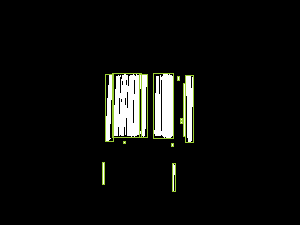On the basis that individual bars in a barcode are tall compared to the largest possible width that any of them can be (which I'll go into more detail about later on), it seems like a reasonable idea to take any areas that are taller than they are wide and expand their width until they become square. That would give us this:We'd then work out which of these "squared off" rectangles overlap (if any) and replace overlapping rectangles with rectangles that cover their combined areas, which would look like this: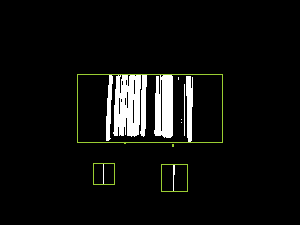The only problem with this is that the combined rectangles extend too far to the left and right of the areas, so we need to trim them down. The will be fairly straightforward because we have the information about what distinct objects there are and each object is just a list of points - so we work out which objects have points within each of the combined bounding areas and then we work out which out of all of the objects for each combined area has the smallest "x" value and smallest "y" value and which have the largest values. That way, we can change the combined bounding areas to only cover actual barcode pixels. Which would leave us with this:That might sound like a lot of complicated work but if we take a bit of a brute force* approach to it then it can be expressed like this:

``````private static IEnumerable<Rectangle> GetOverlappingObjectBounds(
IEnumerable<IEnumerable<Point>> objects)
{
// Translate each "object" (a list of connected points) into a bounding box (squared off if
// it was taller than it was wide)
var squaredOffBoundedObjects = new HashSet<Rectangle>(
objects.Select((points, index) =>
{
var bounds = Rectangle.FromLTRB(
points.Min(p => p.X),
points.Min(p => p.Y),
points.Max(p => p.X) + 1,
points.Max(p => p.Y) + 1
);
if (bounds.Height > bounds.Width)
bounds.Inflate((bounds.Height - bounds.Width) / 2, 0);
return bounds;
})
);

// Loop over the boundedObjects and reduce the collection by merging any two rectangles
// that overlap and then starting again until there are no more bounds merges to perform
while (true)
{
var combinedOverlappingAreas = false;
foreach (var bounds in squaredOffBoundedObjects)
{
foreach (var otherBounds in squaredOffBoundedObjects)
{
if (otherBounds == bounds)
continue;

if (bounds.IntersectsWith(otherBounds))
{
squaredOffBoundedObjects.Remove(bounds);
squaredOffBoundedObjects.Remove(otherBounds);
Math.Min(bounds.Left, otherBounds.Left),
Math.Min(bounds.Top, otherBounds.Top),
Math.Max(bounds.Right, otherBounds.Right),
Math.Max(bounds.Bottom, otherBounds.Bottom)
));
combinedOverlappingAreas = true;
break;
}
}
if (combinedOverlappingAreas)
break;
}
if (!combinedOverlappingAreas)
break;
}

return squaredOffBoundedObjects.Select(bounds =>
{
var allPointsWithinBounds = objects
.Where(points => points.Any(point => bounds.Contains(point)))
.SelectMany(points => points)
.ToArray(); // Don't re-evaluate in the four accesses below
return Rectangle.FromLTRB(
left: allPointsWithinBounds.Min(p => p.X),
right: allPointsWithinBounds.Max(p => p.X) + 1,
top: allPointsWithinBounds.Min(p => p.Y),
bottom: allPointsWithinBounds.Max(p => p.Y) + 1
);
});
}
``````

* (There are definitely more efficient ways that this could be done but since we're only looking at 300px images then we're not likely to end up with huge amounts of data to deal with)

To complete the process, we need to do three more things:

1. Since barcodes are wider than they are tall, we can discard any regions that don't fit this shape (of which there are two in the example image)
2. The remaining regions are expanded a little across so that they more clearly surround the barcode region, rather than being butted right up to it (this will make the barcode reading process a little easier)
3. As the regions that have been identified may well be on a resized version of the source image, they may need to scaled up so that they correctly apply to the source

To do that, we'll start from this code that we saw earlier:

``````var mask = Blur(Normalise(combinedGradients), maxRadiusForGradientBlurring)
``````

.. and expand it like so (removing the "// .. more will go here soon" comment), using the methods above:

``````// Determine how much the image was scaled down (if it had to be scaled down at all)
// by comparing the width of the potentially-scaled-down data to the source image
var reducedImageSideBy = (double)image.Width / greyScaleImageData.Width;

.Where(boundedObject => boundedObject.Width > boundedObject.Height)
.Select(boundedObject =>
{
var expandedBounds = boundedObject;
expandedBounds.Inflate(width: expandedBounds.Width / 10, height: 0);
expandedBounds.Intersect(
Rectangle.FromLTRB(0, 0, greyScaleImageData.Width, greyScaleImageData.Height)
);
return new Rectangle(
x: (int)(expandedBounds.X * reducedImageSideBy),
y: (int)(expandedBounds.Y * reducedImageSideBy),
width: (int)(expandedBounds.Width * reducedImageSideBy),
height: (int)(expandedBounds.Height * reducedImageSideBy)
);
});
``````

The final result is that the barcode has been successfully located on the image - hurrah!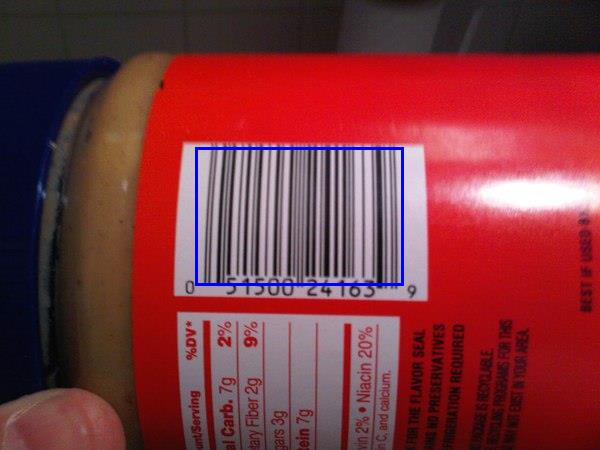With this information, we should be able to extract regions or "sub images" from the source image and attempt to decipher the barcode value in it (presuming that there IS a bar code in it and we haven't got a false positive match).

As we'll see in a moment, the barcode doesn't have to be perfectly lined up - some rotation is acceptable (depending upon the image, up to around 20 or 30 degrees should be fine). The MyFitnessPal app has a couple of fallbacks that I've noticed, such as being able to read barcodes that are upside down or even back to front (which can happen if a barcode is scanned from the wrong side of a transparent wrapper). While I won't be writing code here for either of those approaches, I'm sure that you could envisage how it could be done - the source image data could be processed as described here and then, if no barcode is read, rotated 180 degrees and re-processed and reversed and re-processed, etc..

### How to read a bar code

A barcode is comprised of both black and white bars - so it's not just the black parts that are significant, it is the spaces between them as well.

The format of a barcode is as follows:

1. Three single-width bars (a black one, a white one and another black one) that are used to gauge what is considered to be a "single width"
2. Information for six numbers then appears, where each number is encoded by a sequence of four bars (white, black, white, black) - particular combinations of bar widths relate to particular digits (see below)
3. Another guard section appears with five single width bars (white, black, white, black, white)
4. Six more numbers appear (using the same bar-width-combinations encoding as before but the groups of four bars are now black, white, black, white)
5. A final guard section of three single width bards (black, white, black)

The numbers are encoded using the following system:

`````` Digit      Bar widths

0        3, 2, 1, 1
1        2, 2, 2, 1
2        2, 1, 2, 2
3        1, 4, 1, 1
4        1, 1, 3, 2
5        1, 2, 3, 1
6        1, 1, 1, 4
7        1, 3, 1, 2
8        1, 2, 1, 3
9        3, 1, 1, 2
``````

(Note that every combination of values totals 7 when they added up - this is very helpful later!)

To see what that looks like in the real world, here's a slice of that barcode from the jar of peanut butter with each section and each numberic value identified: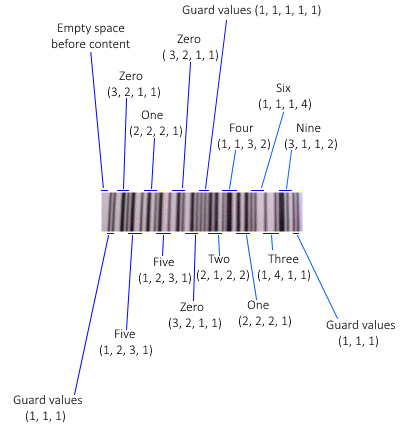(I should point out that the article How does a barcode work? was extremely helpful in the research I did for this post and I'm very grateful to the author for having written it in such an approachable manner!)

Any combination of bar widths that is not found in the table is considered to be invalid. On the one hand, you might think that this a potential loss; the format could support more combinations of bar widths to encode more values and then more data could be packed into the same space. There is an advantage, however, to having relatively few valid combinations of bar widths - it makes easier to tell whether the information being read appears to be correct. If a combination is encountered that seems incorrect then the read attempt should be aborted and retried. The format has existed for decades and it would make sense, bearing that in mind, to prioritise making it easier for the hardware to read rather prioritising trying to cram as much data in there as possible. There is also a checksum included in the numerical data to try to catch any "misreads" but when working with low resolutions or hardware with little computing power, the easier that it is to bail out of a scan and to retry the better.

The way to tackle the reading is to:

1. Convert the sub image to greyscale
2. Create a binary mask so that the darker pixels become 0 and the lighter ones become 1
3. Take a single line across the area
4. Change the individual 1s and 0s into lengths of continuous "runs" of values
• eg. 0001100 would become 3, 2, 2 because there are three 0s then two 1s and then two 0s
5. These runs of values will represent the different sized (black and white) bars that were encountered
• For a larger image, each run length will be longer than for a small image but that won't matter because when we encounter runs of four bar length values that we think should be interpreted as a single digit, we'll do some dividing to try to guess the average size of a single width bar
6. Take these runs of values, skip through the expected guard regions and try to interpret each set of four bars that is thought to represent a digit of the bar code as that digit
7. If successful then perform a checksum calculation on the output and return the value ass a success if it meets expectations
8. If the line couldn't be interpreted as a barcode or the checksum calculation fails then take the next line down and go back to step 4
9. If there are no more lines to attempt then a barcode could not be identified in the image

This processing is fairly light computationally and so there is no need to resize the "may be a barcode" image region before attempting the work. In fact, it's benefical to not shrink it as shrinking it will likely make the barcode section fuzzier and that makes the above steps less likely to work - the ideal case for creating a binary mask is where there is no significant "seepage" of pixel intensity between the black bar areas and the white bar areas. That's not to say that the images have to be crystal clear or perfectly aligned with the camera because the redundancy built into the format works in our favour here - if one line across the image can't be read because it's fuzzy then there's a good chance that one of the other lines will be legible.

60 length values is the precise number that we expect to find - there is expected to be some blank space before the barcode starts (1) and then a guard section of three single-width lines that we use to gauge bar width (3) and then six numbers that are encoded in four bars each (6x4=24) and then a guard section of five single-width lines (5) and then six numbers (6x4=24) and then a final guard region of three single-width bars, giving 1+3+24+5+24+3=60.

There will likely be another section of blank content after the barcode that we ignore

If we don't want to validate the final guard region then we can work with a barcode image where some of the end of cut off, so long as the data for the 12 digits is there; in this case, 57 lengths if the minimum number that we can accept

### Reading the numeric value with code

I'm going to try to present the code in approximately the same order as the steps presented above. So, firstly we need to convert the sub image to greyscale and create a binary mark from it. Then we'll go line by line down the image data and try to read a value. So we'll take this:

``````public static string? TryToReadBarcodeValue(Bitmap subImage)
{
const double threshold = 0.5;

// Black lines are considered 1 and so we set to true if it's a dark pixel (and 0 if light)
var mask = subImage.GetGreyscale().Transform(intensity => intensity < (256 * threshold));
for (var y = 0; y < mask.Height; y++)
{
if (value is object)
return value;
}
return null;
}
``````

.. and the read-each-slice-of-the-image code looks like this:

``````private static string? TryToReadBarcodeValueFromSingleLine(
DataRectangle<bool> barcodeDetails,
int sliceY)
{
if ((sliceY < 0) || (sliceY >= barcodeDetails.Height))
throw new ArgumentOutOfRangeException(nameof(sliceY));

var lengths = GetBarLengthsFromBarcodeSlice(barcodeDetails, sliceY).ToArray();
if (lengths.Length < 57)
{
// As explained, we'd like 60 bars (which would include the final guard region) but we
// can still make an attempt with 57 (but no fewer)
// - There will often be another section of blank content after the barcode that we ignore
// - If we don't want to validate the final guard region then we can work with a barcode
//   image where some of the end is cut off, so long as the data for the 12 digits is
//   there (this will be the case where there are only 57 lengths)
return null;
}

var offset = 0;
var extractedNumericValues = new List<int>();
for (var i = 0; i < 14; i++)
{
if (i == 0)
{
// This should be the first guard region and it should be a pattern of three single-
// width bars
offset += 3;
}
else if (i == 7)
{
// This should be the guard region in the middle of the barcode and it should be a
// pattern of five single-width bars
offset += 5;
}
else
{
var value = TryToGetValueForLengths(
lengths[offset],
lengths[offset + 1],
lengths[offset + 2],
lengths[offset + 3]
);
if (value is null)
return null;
offset += 4;
}
}

// Calculate what the checksum should be based upon the first 11 numbers and ensure that
// the 12th matches it
if (extractedNumericValues.Last() != CalculateChecksum(extractedNumericValues.Take(11)))
return null;

return string.Join("", extractedNumericValues);
}
``````

With the code below, we find the runs of continous 0 or 1 lengths that will represent bars are return that list (again, for larger images each run will be longer and for smaller images each run will be shorter but this will be taken care of later) -

``````private static IEnumerable<int> GetBarLengthsFromBarcodeSlice(
DataRectangle<bool> barcodeDetails,
int sliceY)
{
if ((sliceY < 0) || (sliceY >= barcodeDetails.Height))
throw new ArgumentOutOfRangeException(nameof(sliceY));

// Take the horizontal slice of the data
var values = new List<bool>();
for (var x = 0; x < barcodeDetails.Width; x++)

// Split the slice into bars - we only care about how long each segment is when they
// alternate, not whether they're dark bars or light bars
var segments = new List<Tuple<bool, int>>();
foreach (var value in values)
{
if ((segments.Count == 0) || (segments[^1].Item1 != value))
else
segments[^1] = Tuple.Create(value, segments[^1].Item2 + 1);
}
if ((segments.Count > 0) && !segments.Item1)
{
// Remove the white space before the first bar
segments.RemoveAt(0);
}
return segments.Select(segment => segment.Item2);
}
``````

Now we need to implement the "TryToGetValueForLengths" method that "TryToReadBarcodeValueFromSingleLine" calls. This takes four bar lengths that are thought to represent a single digit in the bar code value (they are not part of a guard region or anything like that). It take those four bar lengths and guesses how many pixels across a single bar would be - which is made my simpler by the fact that all of the possible combinations of bar lengths in the lookup chart that we saw earlier add up to 7.

There's a little flexibility introduced here to try to account for a low quality image or if the threshold was a bit strong in the creation of the binary mask; we'll take that calculated expected width of a single bar and tweak it up or down a little if apply that division to the bar lengths means that we made some of the bars too small that they disappeared or too large and it seemed like the total width would be more than seven single estimated-width bars. There's only a little flexibility here because if we fail then we can always try another line of the image! (Or maybe it will turn out that this sub image was a false positive match and there isn't a bar code in it at all).

``````private static int? TryToGetValueForLengths(int l0, int l1, int l2, int l3)
{
if (l0 <= 0)
throw new ArgumentOutOfRangeException(nameof(l0));
if (l1 <= 0)
throw new ArgumentOutOfRangeException(nameof(l1));
if (l2 <= 0)
throw new ArgumentOutOfRangeException(nameof(l2));
if (l3 <= 0)
throw new ArgumentOutOfRangeException(nameof(l3));

// Take a guess at what the width of a single bar is based upon these four values
// (the four bars that encode a number should add up to a width of seven)
var raw = new[] { l0, l1, l2, l3 };
var singleWidth = raw.Sum() / 7d;
var adjustment = singleWidth / 10;
var attemptedSingleWidths = new HashSet<double>();
while (true)
{
var normalised = raw.Select(x => Math.Max(1, (int)Math.Round(x / singleWidth))).ToArray();
var sum = normalised.Sum();
if (sum == 7)
return TryToGetNumericValue(normalised, normalised, normalised, normalised);

if (sum > 7)
else
if (attemptedSingleWidths.Contains(singleWidth))
{
// If we've already tried this width-of-a-single-bar value then give up -
// it doesn't seem like we can make the input values make sense
return null;
}
}

static int? TryToGetNumericValue(int i0, int i1, int i2, int i3)
{
var lookFor = string.Join("", new[] { i0, i1, i2, i3 });
var lookup = new[]
{
// These values correspond to the lookup chart shown earlier
"3211", "2221", "2122", "1411", "1132", "1231", "1114", "1312", "1213", "3112"
};
for (var i = 0; i < lookup.Length; i++)
{
if (lookFor == lookup[i])
return i;
}
return null;
}
}
``````

Finally we need the CalculateChecksum method (as noted in the code, there's a great explanation of how to do this in wikipedia) -

``````private static int CalculateChecksum(IEnumerable<int> values)
{
if (values == null)
throw new ArgumentNullException(nameof(values));
if (values.Count() != 11)
throw new ArgumentException("Should be provided with precisely 11 values");

// See https://en.wikipedia.org/wiki/Check_digit#UPC
var checksumTotal = values
.Select((value, index) => (index % 2 == 0) ? (value * 3) : value)
.Sum();
var checksumModulo = checksumTotal % 10;
if (checksumModulo != 0)
checksumModulo = 10 - checksumModulo;
return checksumModulo;
}
``````

With this code, we have executed all of the planned steps outlined before.

It should be noted that, even with the small amount of flexibility in the "TryToGetValueForLengths" method, in the peanut butter bar code example it requires 15 calls to "GetBarLengthsFromBarcodeSlice" until a bar code is successfully matched! Presumably, this is because there is a little more distortion further up the bar code due to the curve of the jar.

That's not to say, however, that this approach to bar reading is particularly fussy. The redundancy and simplicity, not to mention the size of the average bar code, means that there is plenty of opportunity to try reading a sub image in multiple slices until one of them does match. In fact, I mentioned earlier that the barcode doesn't have to be perfectly at 90 degrees in order to be interpretable and that some rotation is acceptable. This hopefully makes some intuitive sense based upon the logic above and how it doesn't matter how long each individual bar code line is because they are averaged out - if a bar code was rotated a little and then a read was attempted of it line by line then the ratios between each line should remain consistent and the same data should be readable.

To illustrate, here's a zoomed-in section of the middle of the peanut butter bar code in the orientation shown so far: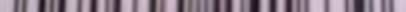If we then rotate it like this: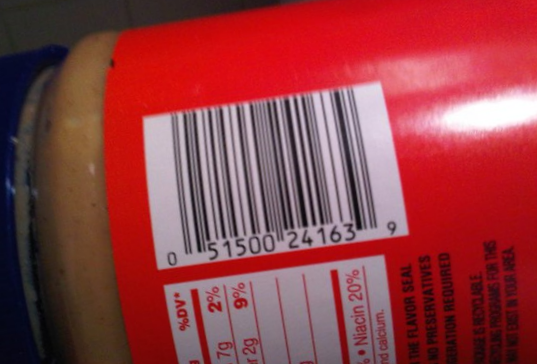.. then the code above will still read the value correctly because a strip across the rotated bar code looks like this: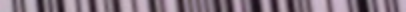Hopefully it's clear enough that, for each given line, the ratios are essentially the same as for the non-rotated strip:To get a reading from an image that is rotated more than this requires a very clear source image and will still be limited by the first stage of processing - that tried to find sections where the horizontal image intensity changed with steep gradients but the vertical intensity did not. If the image is rotated too much then there will be more vertical image intensity differences encountered and it is less likely to identify it as a "maybe a bar code" region.

(Note: I experimented with rotated images that were produced by an online barcode generator and had more success - meaning that I could rotate them more than I could with real photographs - but that's because those images are generated with stark black and white and the horizontal / vertical intensity gradients are maintained for longer when the image is rotated if they start with such a high level of clarity.. I'm more interested in reading values from real photographs and so I would suggest that only fairly moderate rotation will work - though it would still be plenty for an MyFitnessPal-type app that expects the User to hold the bar code in roughly the right orientation!)

### Tying it all together

We've looked at the separate steps involved in the whole reading process, all that is left is to combine them. The "GetPossibleBarcodeAreasForBitmap" and "TryToReadBarcodeValue" methods can be put together into a fully functioning program like this:

``````static void Main()
{
using var image = new Bitmap("Source.jpg");

var barcodeValues = new List<string>();
foreach (var area in GetPossibleBarcodeAreasForBitmap(image))
{
using var areaBitmap = new Bitmap(area.Width, area.Height);
using (var g = Graphics.FromImage(areaBitmap))
{
g.DrawImage(
image,
destRect: new Rectangle(0, 0, areaBitmap.Width, areaBitmap.Height),
srcRect: area,
srcUnit: GraphicsUnit.Pixel
);
}
if (valueFromBarcode is object)
}

if (!barcodeValues.Any())
Console.WriteLine("Couldn't read any bar codes from the source image :(");
else
{
Console.WriteLine("Read the following bar code(s) from the image:");
foreach (var barcodeValue in barcodeValues)
Console.WriteLine("- " + barcodeValue);
}

Console.WriteLine();
Console.WriteLine("Press [Enter] to terminate..");